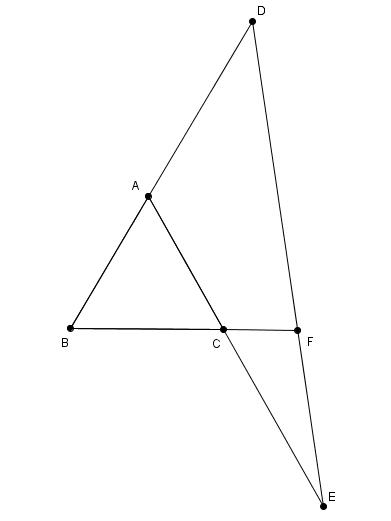# Extensions of a triangle

Geometry Level 4Let be $ABC$ an equilateral triangle of side $1$. $D$ and $E$ points on the extension of $AB$ and $AC$, respectively such that$AD=CE=1$ . $F$ is the point of interseccion between $DE$ and $BC$ extension.

Find the length $CF$ to 3 decimal places.

×